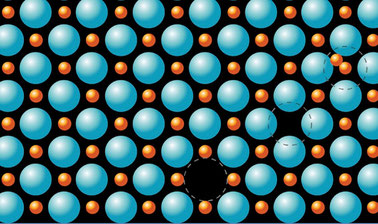# Structure of Materials

Discover the structure of the materials that make up our modern world and learn how this underlying structure influences the properties and performance of these materials.## There is one session available:

After a course session ends, it will be archived.
Estimated 16 weeks
6–8 hours per week
Self-paced

Structure determines so much about a material: its properties, its potential applications, and its performance within those applications. This course from MIT’s Department of Materials Science and Engineering explores the structure of a wide variety of materials with current-day engineering applications.

The course begins with an introduction to amorphous materials. We explore glasses and polymers, learn about the factors that influence their structure, and learn how materials scientists measure and describe the structure of these materials.

Then we begin a discussion of the crystalline state, exploring what it means for a material to be crystalline, how we describe directions in a crystal, and how we can determine the structure of crystal through x-ray diffraction. We explore the underlying crystalline structures that underpin so many of the materials that surround us. Finally, we look at how tensors can be used to represent the properties of three-dimensional materials, and we consider how symmetry places constraints on the properties of materials.

We move on to an exploration of quasi-, plastic, and liquid crystals. Then, we learn about the point defects that are present in all crystals, and we will learn how the presence of these defects lead to diffusion in materials. Next, we will explore dislocations in materials. We will introduce the descriptors that we use to describe dislocations, we will learn about dislocation motion, and will consider how dislocations dramatically affect the strength of materials. Finally, we will explore how defects can be used to strengthen materials, and we will learn about the properties of higher-order defects such as stacking faults and grain boundaries.

### At a glance

• Institution: MITx
• Subject: Engineering
• Level: Intermediate
• Prerequisites:
• University-level chemistry
• Single-variable calculus
• Some basic linear algebra
• Language: English
• Associated programs:

# What you'll learn

Skip What you'll learn
• How we characterize the structure of glasses and polymers
• The principles of x-ray diffraction that allow us to probe the structure of crystals
• How the symmetry of a material influences its materials properties
• The properties of liquid crystals and how these materials are used in modern display technologies
• How defects impact numerous properties of materials—from the conductivity of semiconductors to the strength of structural materials

# Syllabus

Skip Syllabus

Part 1: An Introduction to Materials Science

• States of matter and bonding

Part 2: Descriptors

• Descriptors: concept and function
• Free volume
• Pair distribution function

Part 3: Glasses

• Glass processing methods
• Continuous network model
• Network modifiers

Part 4: Polymers

• Random walk model
• Chain-to-chain end distance
• Order and disorder in polymers

Part 5: An Introduction to the Crystalline State

• Translational symmetry
• The crystalline state in 2D
• The crystalline state in 3D

Part 6: Real and Reciprocal Space

• Miller indices
• Real space
• Reciprocal space

Part 7: X-Ray Diffraction

• Bragg’s Law
• Diffraction examples

Part 8: Symmetry in 2D Crystals

• Translation, mirror, glide and rotation symmetry

Part 9: Point groups in 2D

• Allowed rotational symmetries in crystals

The 10 2D point groups

• An introduction to crystallographic notation

Part 10: Plane groups in 2D

• The five 2D lattice types
• The 17 plane groups in 2D

Part 11: Symmetry in 3D Crystals

• Inversion, Roto-Inversion, and Roto-reflection
• Screw symmetry

Part 12: 3D Space Point groups

• Space point groups
• Stereographic projection

Part 13: 3D Space Groups

• Crystal lattices
• Space groups

Part 14: An Introduction to Tensors

• Symmetry constraints on materials properties
• Coordinate transformation

Part 15: Quasi, Plastic, and Liquid Crystals

• Quasi crystals
• An introduction to plastic and liquid crystals
• Liquid crystal descriptors
• Liquid crystal applications

Part 16: Introduction to Point Defects

• Thermodynamics of point defects
• Vacancies, interstitials, solid solutions and nonequilibrium defects

Part 17: Ionic Point Defects & Diffusion

• Kröger-Vink notation
• Extrinsic defects
• Diffusion

Part 18: Dislocations and Deformation

• Intro d shear stress

Part 19: Strengthening & Surface Energy

• Strengthening Mechanisms
• Surface free energy
• Wulff shape

Part 20: 2-Dimensional Defects

• Surface defects
• Stacking faults
• Grain boundaries
• Surface reconstruction
• Linear defects in liquid crystals